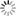# Episode List

OR

### Season 1

18 Sep. 1954
First CaseIn this debut episode, lawyer Willa Dodger (nicknamed WILLY) finally gets her first case, defending the owner of a dog. The dog is accused of scaring a farmer's sheep, and of note is the fact that the dog catcher is portrayed by a very young Aaron Spelling. Needless to say, Willy wins her very first case, kicking-starting her law career.

25 Sep. 1954
Get Charlie's GoatKnow what this is about?
Be the first one to add a plot.

2 Oct. 1954
Operation StocksKnow what this is about?
Be the first one to add a plot.

9 Oct. 1954
New York ColleagueKnow what this is about?
Be the first one to add a plot.

16 Oct. 1954
Willy Chooses Not to RunKnow what this is about?
Be the first one to add a plot.

23 Oct. 1954
Willy's CommissionKnow what this is about?
Be the first one to add a plot.

30 Oct. 1954
Willy and the Crook JuliusKnow what this is about?
Be the first one to add a plot.

6 Nov. 1954
Willy Waves the FlagKnow what this is about?
Be the first one to add a plot.

13 Nov. 1954
Water Witch CaseKnow what this is about?
Be the first one to add a plot.

20 Nov. 1954
Job OfferKnow what this is about?
Be the first one to add a plot.

27 Nov. 1954
The French HatKnow what this is about?
Be the first one to add a plot.

4 Dec. 1954
Willy and Muller vs. MullerKnow what this is about?
Be the first one to add a plot.

11 Dec. 1954
The SubstitutionKnow what this is about?
Be the first one to add a plot.

18 Dec. 1954
Papa's BirthdayKnow what this is about?
Be the first one to add a plot.

25 Dec. 1954
The Fate of the TheatreKnow what this is about?
Be the first one to add a plot.

1 Jan. 1955
The Charity BenefitKnow what this is about?
Be the first one to add a plot.

8 Jan. 1955
Willy and L'Affaire Paul RevereKnow what this is about?
Be the first one to add a plot.

15 Jan. 1955
Willy and Aunt Cora's BeauCora's new boyfriend seems like a nice fellow and the family approves. Imagine their surprise while watching TV in which the man stars in a real crime drama..

22 Jan. 1955
Willy and Grandpa's PianoKnow what this is about?
Be the first one to add a plot.

29 Jan. 1955
Puppy LoveKnow what this is about?
Be the first one to add a plot.

5 Feb. 1955
Willy and the Mystery PackageKnow what this is about?
Be the first one to add a plot.

12 Feb. 1955
The Daniel Boone CaseKnow what this is about?
Be the first one to add a plot.

19 Feb. 1955
Willy's NephewKnow what this is about?
Be the first one to add a plot.

26 Feb. 1955
Willy Doubles in BrassKnow what this is about?
Be the first one to add a plot.

5 Mar. 1955
Willy and the Farewell DanceKnow what this is about?
Be the first one to add a plot.

12 Mar. 1955
New York BoundKnow what this is about?
Be the first one to add a plot.

19 Mar. 1955
Willy and the Kate Fleming CaseKnow what this is about?
Be the first one to add a plot.

26 Mar. 1955
Willy and Hansel and GretelKnow what this is about?
Be the first one to add a plot.

7 Apr. 1955
Willy's New York NeighborsKnow what this is about?
Be the first one to add a plot.

14 Apr. 1955
Papa's Hot TipKnow what this is about?
Be the first one to add a plot.

21 Apr. 1955
Willy and the CounterfeitersKnow what this is about?
Be the first one to add a plot.

28 Apr. 1955
Willy's New DressKnow what this is about?
Be the first one to add a plot.

5 May 1955
Willy Saves Harvey from FraudThe library fund for Renfrew, New Hampshire, is accidentally spent on a fund for a skyscraper in New York City. Willy must find a way to rectify the mistake and save Harvey from going to jail for fraud.

12 May 1955
The Big FightKnow what this is about?
Be the first one to add a plot.

19 May 1955
Willy's PaintingKnow what this is about?
Be the first one to add a plot.

26 May 1955Know what this is about?
Be the first one to add a plot.

2 Jun. 1955
Willy and El FlamencoKnow what this is about?
Be the first one to add a plot.

9 Jun. 1955
Willy and Eccentric HenriettaKnow what this is about?
Be the first one to add a plot.

16 Jun. 1955
Willy and Hurricane HarveyKnow what this is about?
Be the first one to add a plot.

Season 1